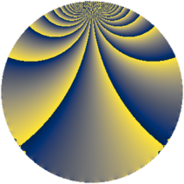Properties

 Label 432.1.gLevel $432$ Weight $1$ Character orbit 432.g Rep. character $\chi_{432}(271,\cdot)$ Character field $\Q$ Dimension $2$ Newform subspaces $1$ Sturm bound $72$ Trace bound $0$

Related objects

Defining parameters

 Level: $$N$$ $$=$$ $$432 = 2^{4} \cdot 3^{3}$$ Weight: $$k$$ $$=$$ $$1$$ Character orbit: $$[\chi]$$ $$=$$ 432.g (of order $$2$$ and degree $$1$$) Character conductor: $$\operatorname{cond}(\chi)$$ $$=$$ $$4$$ Character field: $$\Q$$ Newform subspaces: $$1$$ Sturm bound: $$72$$ Trace bound: $$0$$

Dimensions

The following table gives the dimensions of various subspaces of $$M_{1}(432, [\chi])$$.

Total New Old
Modular forms 22 2 20
Cusp forms 4 2 2
Eisenstein series 18 0 18

The following table gives the dimensions of subspaces with specified projective image type.

$$D_n$$ $$A_4$$ $$S_4$$ $$A_5$$
Dimension 2 0 0 0

Trace form

 $$2 q + O(q^{10})$$ $$2 q + 2 q^{13} - 2 q^{25} - 2 q^{37} - 4 q^{49} - 2 q^{61} + 2 q^{73} + 2 q^{97} + O(q^{100})$$

Decomposition of $$S_{1}^{\mathrm{new}}(432, [\chi])$$ into newform subspaces

Label Dim. $$A$$ Field Image CM RM Traces $q$-expansion
$a_{2}$ $a_{3}$ $a_{5}$ $a_{7}$
432.1.g.a $2$ $0.216$ $$\Q(\sqrt{-3})$$ $D_{6}$ $$\Q(\sqrt{-3})$$ None $$0$$ $$0$$ $$0$$ $$0$$ $$q+(\zeta_{6}+\zeta_{6}^{2})q^{7}+q^{13}+(-\zeta_{6}-\zeta_{6}^{2}+\cdots)q^{19}+\cdots$$

Decomposition of $$S_{1}^{\mathrm{old}}(432, [\chi])$$ into lower level spaces

$$S_{1}^{\mathrm{old}}(432, [\chi]) \cong$$ $$S_{1}^{\mathrm{new}}(144, [\chi])$$$$^{\oplus 2}$$